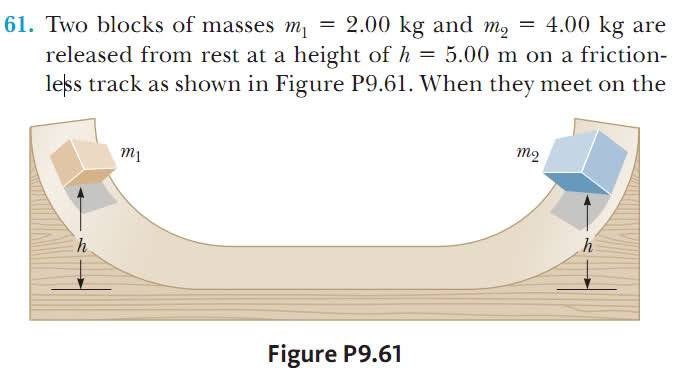# Linear momentum and velocity direction

Hi everyone
When using the concept of the conservation of the linear momentum ΣPi = ΣPf to solve a problem, should I consider the the direction of the velocity? For Example, the following problemwhich one of the following equations is correct?
m1v0+m2v0=m1v1+m2v2
or
m1v0-m2v0=-m1v1+m2v2
note: both of the blocks have the same intial velocity (v0) because the surface is frictionless.

cjl
Momentum is a vector quantity, so you absolutely do have to pay attention to the direction when solving problems with conservation of momentum.

Doc Al
Mentor
When using the concept of the conservation of the linear momentum ΣPi = ΣPf to solve a problem, should I consider the the direction of the velocity?
As cjl already stated, you must take direction into account.

which one of the following equations is correct?
m1v0+m2v0=m1v1+m2v2
or
m1v0-m2v0=-m1v1+m2v2
note: both of the blocks have the same intial velocity (v0) because the surface is frictionless.
Careful here. You're really using v0 as the initial speed; the initial velocities are +v0 and -v0. After the collision I would not make any assumptions about the directions; just let v1 and v2 be the velocities, which you'll solve for.

Thank you very much guys :)